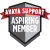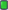Thread: Cms r14 View Single Post
#1
 sciskAspiring MemberJoin Date: May 2016 Posts: 2Cms r14

Dear all,

I would like to ask for support in below case. I tried two different reports:

REPORT A
select
magent.LOGID, magent.LOGID, magent.sum(TOTAL_ACDCALLS)+sum(AUXINCALLS)+sum(ACW INCALLS)+sum(EXT_CALL_OUT), AVG_AGENT_TALK_TIME FROM magent

where
ROW_DATE = [Date:] and ACD=\$acd and LOGID in (select value from agroups where acd_no=\$acd and item_name = [Agent Group:] ) GROUP BY 1, 2, 4 ORDER BY magent.LOGID

REPORT B
select
magent.LOGID, magent.sum(TOTAL_ACDCALLS)+sum(AUXINCALLS)+sum(ACW INCALLS)+sum(EXT_CALL_OUT), AVG_AGENT_TALK_TIME FROM magent

where
ROW_DATE = [Month Starting:] and ACD=\$acd and LOGID in (select value from agroups where item_name = [Agent Group:] and acd_no=\$acd) GROUP BY 1, 3

In both cases data per Agent are not agregated - it means one Agent with 3 skills has 3 rows. Is it because i use AVG data or because there is this sum of magent.sum(TOTAL_ACDCALLS)+sum(AUXINCALLS)+sum(ACW INCALLS)+sum(EXT_CALL_OUT)